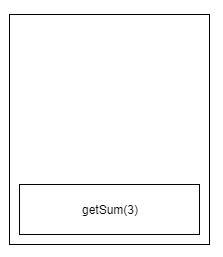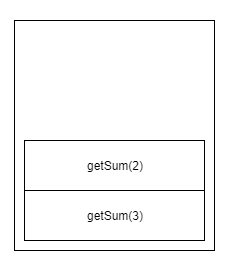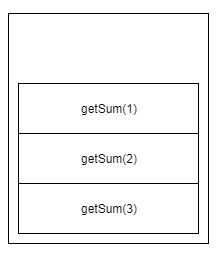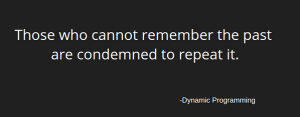Recursion is not hard, Here is the right way to think!

• January 2, 2019
• 2Shalitha Suranga

Software Engineering Intern
Open Source Developer | Apache Committer and PMC Member

Recursion is one of most useful strategies which can be used in algorithm design. Therefore understanding how recursion behaves in computer programs is mandatory for all computer engineers. Recursion is not hard, whereas thinking recursively might be confusing in some cases. The recursive algorithm has considerable advantages over identical iterative algorithm such as having fewer code lines and reduced use of data structures. But, well-known drawbacks of recursion are high memory usage and slow running time since it uses function call stack. Furthermore, every recursive solution can be converted into an identical iterative solution using the stack data structure, and vice versa.

Function

Function(aka method, routine or procedure) in computer programming is almost similar to a function in mathematics. Generally, It has an input and an output. Also, there is a logical body to make output using input(s). A function can be called from other functions and a function can be called from itself as well.

Recursion

“Recursion is a process in which a function calls itself as a subroutine.”

– TechTerms

If the same process needs to be applied for different inputs inside the same function, simply a function call can be used inside the same function. Thus, for this situation, we name as “recursion”.

Suppose you are given a simple question at a tech interview.

[Q1]: Write a function that prints 1 to 10

function printNumbers()
for i in 1 to 10
print i

printNumbers() // call printNumbers()

Here we are using the iterative approach by going through a loop. Suppose the interviewer asks another question

[Q2]: Redo [Q1] without using loop structures

Think about the loop, it simply does print i –> i+1 –> repeat until i reach 11. So it is doing the same thing over and over. So let’s put stuff to a function

function printNumbers(n)
print n
n = n + 1

So, the above thing will happen again but with different n

function printNumbers(n)
print n
n = n + 1
printNumbers(n) // do that again

printNumbers(1) // call printNumbers with input 1

But there is an issue.n will take values [1,2,3……….10,11,….] and this will take infinite time to finish theoretically. Whereas the well-known stack overflow error will stop this. Therefore we need to mention where to stop these function call chain.

function printNumbers(n)
if n == 11
return
print n
n = n +1
printNumbers(n) // do that again

printNumbers(1) // call printNumbers with input 1

When n is 11 we are not going to call printNumbers again but we simply exit the function. This is what we call a base case of a recursive function. In other words, it is the stopping condition.

Recursion + Return

Above scenario is obviously simple for everyone because it is very similar to iterative way. Importantly it doesn’t use the real usage of the function call stack. Suppose the same interviewer from the previous section asked another question

[Q3]: Write a recursive function which calculates the sum of 1 to n numbers when n is given.

Eg : f(n=3) should return 6 (1 + 2 + 3 = 6)

So, this might be a bit confusing to find what is repeating. So take some sample values starting from the minimum.

f(1) = 1 = 1
f(2) = 2 + 1 = 3
f(3) = 3 + 2 + 1 = 6
f(4) = 4 + 3 + 2 + 1 = 10

Reuse functions instead of elements from the right side

f(4) = 4 + 3 + 2 + f(1)
= 4 + 3 + f(2)
= 4 + f(3)

Write for n

f(n) = n + f(n-1)

This is the recursive definition. Let’s put all together to a function

function getSum(n)
if n == 1
return 1
return n + getSum(n - 1)

getSum(3) // returns 6

Behind the scenes

A general computer program has 3 major parts in its memory model as per below.

Text: contains instructions of the program

Heap: Dynamic memory area where all dynamic memory allocations happen

Stack: Stores local variables of functions, arguments and routine information (pointers and addresses). Recursion strategy uses this call stack data structure.

Suppose we are calling getSum(3)

Step 1: getSum(3) stack frame is pushed to the function call stackStep 2: getSum(3) executes n + getSum(2) we know n is 3 but we don’t know what getSum(2) is, it is also pushed to the function call stack.Step 3: getSum(2) executes n + getSum(1) we know n is 2 but we don’t know still what getSum(1) is, it is also pushed to function stack.Step 4: getSum(1) is 1. So we need to reverse the function call chain since this is a stack data structure.

Step 5: getSum(1) is popped from the stack and return value is used in n + getSum(1). So getSum(2) is 3Step 6: getSum(2) is popped from the stack and return value is used in n + getSum(2). So getSum(3) is 3Step 5: getSum(3) is popped from the stack and the return value is displayed as the output which is 6.

What makes recursion confusing?

The key reason is that we are looking at the same function with different values of local variables. It is very important to make sure which input is currently being used when you are analyzing a recursive function. Thus, the stack is a LIFO(Last In First Out) structure so recursion chain is reversed in order to take the value of everything. When recursion happens it’s good to consider recurred function as another function which is having different local variables.

When there is a recursive call, it will be executed by holding the rest of the statements which reside after the recursive call. This is known as head recursion

Eg:

function func(n):
if n == 0:
return
f(n - 1)
print(n)

func(10) // call func with input 10

print(n) will be executed after the execution of f(n -1)  function call chain. In other words, all the print(n) statements are in hold mode until the whole future recursive chain reverse back. Therefore above pseudo code will print 1 to 10, not 10 to 1.

Recursive algorithm design steps

1.  First of all, find what is repeating. If found put stuff into a function(eg: Q2 ).

2. Otherwise find the relationship of nth answer and (n-1)th answer (eg: Q3). Then construct a recursive definition using a mathematical function.

3. Add a base case(s)(Stopping condition for recursion to avoid stack overflow error)

Conclusion

Recursion is a very useful technique in tree-related algorithms. Divide and Conquer strategy also uses recursion. A key advantage of recursion is that it is remembering the past with the help of call stack structure. Thus those sub problems which can be solved using recursive strategy may be overlapped on each other. Therefore some kind of cache can be introduced in order to reduce excessive function calls which leads to the higher time complexity of the specific algorithm. Dynamic Programming(aka DP) is known as this caching mechanism.Happy Coding! 💪

•John Doe January 2, 2019

Recursion is not always the fastest way…

function getSum(n):
return (n*n+n)/2

•